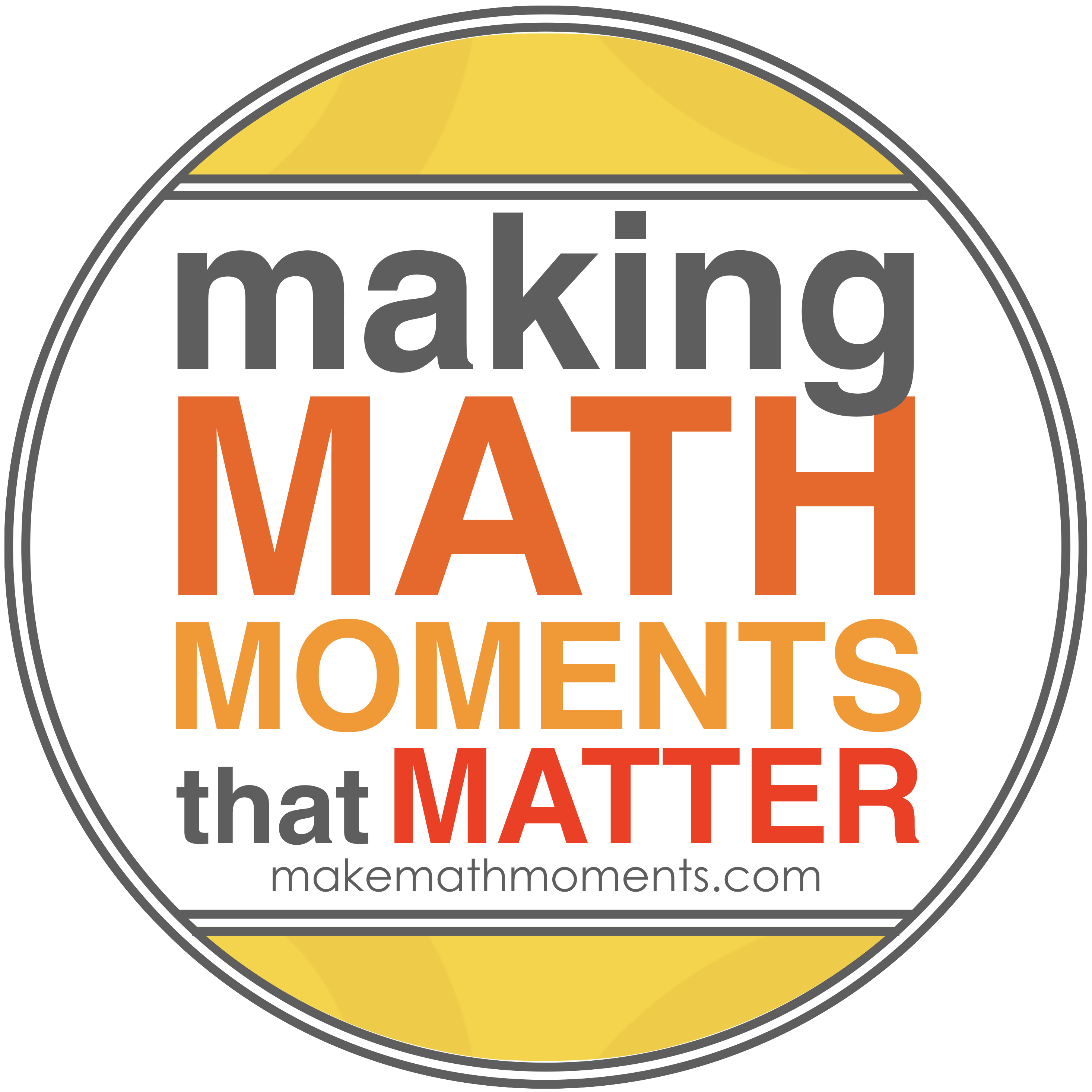# U2 – Transformations

U2 Transformations

 Chapter 2 Transformations of Functions Day Topic/ Section Teacher Files/Instructions Student Work 2.1 Functions & Equivalent Algebraic representations Pg. 83 # 3 – 6, 11, 15 2.2 Skills you will need – Operations with rational expressions Day 1 – Multiplying & Dividing rational expressions Day 2 – Adding and subtracting rational expressions Day 1 – Pg. 94 # 1 – 6 Day 2 – Pg. 94 # 7 – 9, 11a, 19 2.3 Horizontal & Vertical Transformations Using graphing calculators and graph paper, discuss horizontal & Vertical transformations. Pg. 102 # (2 – 5) ac, 6dgj, 8 2.4 Reflections of Functions Using graphing calculators and graph paper discuss reflections. Pg. 110 # (1 – 3)ef, 5and graph: 2.5 Stretches of Functions Using graphing calculators and graph paper discuss stretches. Pg. 120 # 3, 4bcde, 6 2.6 Combinations of Transformations Apply all transformations to the basic graphs. Use a table of values and mapping notation to help. Pg. 129 #(1, 3)ac, 5cd, 7, 12 2.7 Inverse of a function Quiz + Inverse of a function Pg. 138 # (1 – 7)ac, 8, 11, 12, 18 Review TIPS/Test